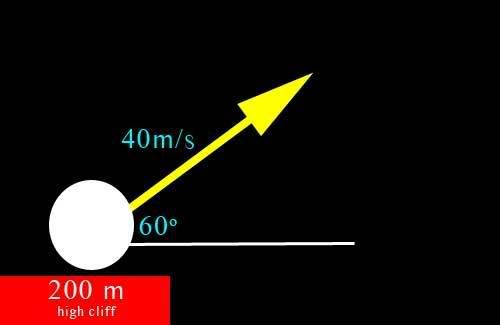# Golf ball problem (velocity, max height, hang time) (1 Viewer)

### Users Who Are Viewing This Thread (Users: 0, Guests: 1)

#### aquaorange

"golf ball problem" (velocity, max height, hang time)

Okay. I'm having a really hard time with this. I know how to start the problem, but after that I go nuts, and literally start pulling out my hair. There is no book I can look in for reference, as this part is teacher supplemented.

The given picture/information is this:And I need to find:

velocity of x at zero time:
velocity of y at zero time:
maximum height (from ground, not cliff):
hang time:
velocity of x after "a" time:
velocity of y after "a" time:

Now, I can find Vx at zero (20m/s), and Vy at zero (34.6m/s). I also know that Vx at any time is going to remain the same (20m/s), but then I'm lost for maximum height, hang time, and Vy at "a" time. Gravity is taken into account, but not air resistance. I know that it loses -9.8m/s going up due to gravity, and +9.8m/s going down due to gravity. And I tried doing that a long way, subtracting 9.8 from 34.6, subtracting 9.8 form that answer, etc., and counting how many times to get a seconds number, but then I didn't know how to figure out when I got down to 5.2 and subtracting 9.8 from that. I really have no clue how far up it goes, or how to find anything past making the triangle to find the forces using sine/cosine/tangent.

I'm not sure if there is a formula or multiple formulas to figure this information out. That's what I think I'm missing to get the rest of the information.

Homework Helper
The formula which will be of great use to you is the displacement formula. For the x direction, you have $$x(t) = x_{0} + v_{0x}t$$, and, for the y direction, $$y(t) = y_{0} + v_{0y}t - \frac{1}{2}gt^2$$. You should be able to solve the problem now.

### The Physics Forums Way

We Value Quality
• Topics based on mainstream science
• Proper English grammar and spelling
We Value Civility
• Positive and compassionate attitudes
• Patience while debating
We Value Productivity
• Disciplined to remain on-topic
• Recognition of own weaknesses
• Solo and co-op problem solving